# FUNCTIONAL CALCULATION OF PROCESS FLOWS’ PARAMETERS IN SPREADSHEETS

Kontsevoy Sergey1, Kontsevoy Andrey1
1Igor Sikorsky Kyiv Polytechnic Institute, Faculty of Chemical Technology, Associate Professor

Abstract
A functional approach to the calculation of process flows in chemical technology is presented. It based on the calculation of apparatuses (mixers, splitters, and reactors) as functions realized in the VBA-Excel. The iterative possibilities of the Excel program are used for calculation of technological schemes with recirculation of flows. As an example, the calculation of one and two-stage reverse osmosis installations are used. For this approach, the elementary knowledge of VBA language is required at the level of the calculations for three types of apparatus and their combinations in user functions. At the same time, visualization of connections between functions on the Excel sheet, simplifies the organization of calculations, especially for beginners.

Category: 05.00.00 Technical sciences

Article reference:
Kontsevoy S., Kontsevoy A. Functional calculation of process flows' parameters in spreadsheets // Modern scientific researches and innovations. 2018. № 5 [Electronic journal]. URL: https://web.snauka.ru/en/issues/2018/05/86532View this article in Russian

Calculation of any chemical-technological scheme is an obligatory element both in the analysis of existing schemes and while the synthesis of new ones. At present, there is no single approach for calculating schemes of any complexity level. The complexity of chemical technologies is determined both by the number of structural elements (devices) and the presence of recycles of technological flows.

Such types of complexity were characteristic of the pre-computer era, and at the present time, neither a large number of elements in the scheme nor the presence of recyclings is a serious obstacle to the calculation of the parameters of technological schemes. Therefore, consider these “problems” in general and on specific examples.

Apparatus as a function
The most actively functional approach  is used in modern programming languages (Erlang, Haskell, Clojure, Scala…). A characteristic feature of this approach is the use of functions as “black boxes”. This means that the internal logic of the functions is hidden from the environment, and the interaction between them is carried out through their inputs and outputs. That is, the outputs of some functions (the results of their calculation) are inputs (parameters) of other functions.
In the same sense, we can also consider apparatuses in chemical-technological schemes, since this corresponds to the actual state in them. Each device interacts with the other only by taking and giving out streams of matter passing through them.

The peculiarity of the proposed solution is the use of the non-functional programming language VBA in the programming environment of Excel which is reactive  in the sense that any changes in the values in the cells result in automatic recalculation of all dependent cells. In this environment, any VBA functions interact with each other through the I / O cells of the Excel sheet and in no other way. In fact, the ability to define user-defined functions in VBA is sufficient to implement the proposed approach. That is, any programming language that supports the definition of functions can be used. The second important feature of the proposed programming environment is the possibility of implementing iterative calculations, which avoids programming cycles in the VBA language by hand.

Another important feature of the calculation’s organization on the Excel sheet is its visibility. Excel sheet allows displaying the technological scheme in the usual way on it, while in the place of the displayed devices will be placed calls of the corresponding functions. The result of such a calculations’ organization will be a “live” scheme where changing the input parameters leads to a change in all output parameters.

Calculation of the reverse osmosis installation
As it was mentioned above, all apparatuses in chemical technology are divided into three types (and their combinations) from the point of view of calculations based on the material balance .
To illustrate the basics of the proposed approach presented the reverse osmosis (RO) unit with concentrate recycling that is selected since it consists of just these three variants and one of their combinations. The membrane is a reactor and a splitter at the same time: water desalination occurs in it and the input stream is divided into two: permeate and concentrate. The splitter separates the concentrate stream into recycling and sewage, and the mixer mixes the recycle stream with the raw water and feeds this mixture to the inlet of the membrane.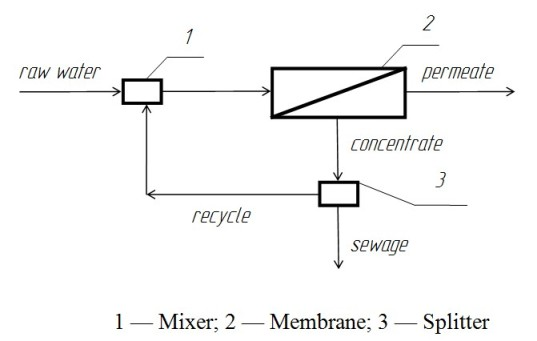Figure 1. Scheme of the single-stage RO unit with concentrate recycling

The main objective of this calculation is to determine the allowable degree of recycling (Xr), which is defined as the ratio of the recycling flow (Gr) to the concentrate flow after the membrane (Gk). It is known that exceeding the permissible level of recycling leads to the formation of deposits on the surface of the membrane. The parameters Xr and Gk are the input parameters of the splitter and the corresponding function (fig. 2), and the recycle flow and sewage flow rates (Gs) are the output parameters (the results of the function calculation). Obviously, TDS (total dissolved solids) of water does not change on the splitter and therefore this parameter does not use for this function.

Equations for the function of the mixer are derived from the material balances of water flows and the flow rate of dissolved solids per hour. The input parameters are the water’s flow rate and TDS of the recycling water, and the flow rate of raw water and water at the outlet are the output parameters for the mixer (Gv). The output parameters are the flow of the raw water (Gi) and TDS of water at the outlet from the mixer.
It should be noted that physically the input parameter Gi became the output parameter for the function due to its dependence on the recycle flow rate since the flow rate at the outlet from the mixer is a constant and is determined by the performance of the membrane. For example, a reduction of the recycling rate (Xr) results in a reduction of the recycle flow, which will lead to an increase in the flow of raw water while the water flow rate at the outlet from the mixer will remain constant.

When organizing the calculations of the membrane, all the principles of material balances for water flow rates and water’s TDS (in form G*TDS) are taken into account. In addition, the parameters of the membrane itself are used – the permeate output (Xp) and the water desalination degree (Xd).

The functions developed in VBA are shown in Figure 2.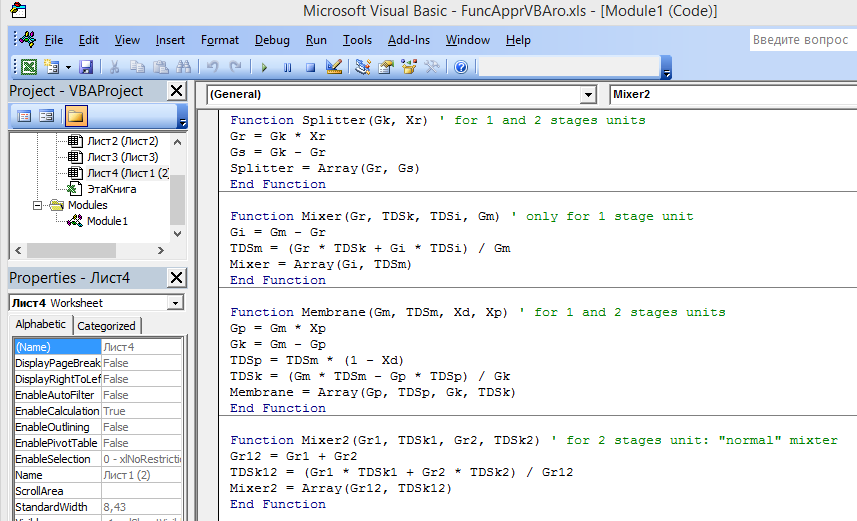Figure 2. The  VBA functions

Note that to get access to user-defined functions via the standard menu, one have to place them in a module that is not present in the spreadsheet by default.

Assembling the functions in Excel

The assembly of functions on the Excel sheet with the recycling scheme can begin with any element, but we started with the membrane, continued with the separator and ended with a mixer (fig. 3).  Note that after displaying one calculation result for the used functions with 2 or more results, it is necessary to select (from left to right) the number of cells equal to the number of return values, then press the F2 key, and then simultaneously press the Ctrl, Shift and Enter keys.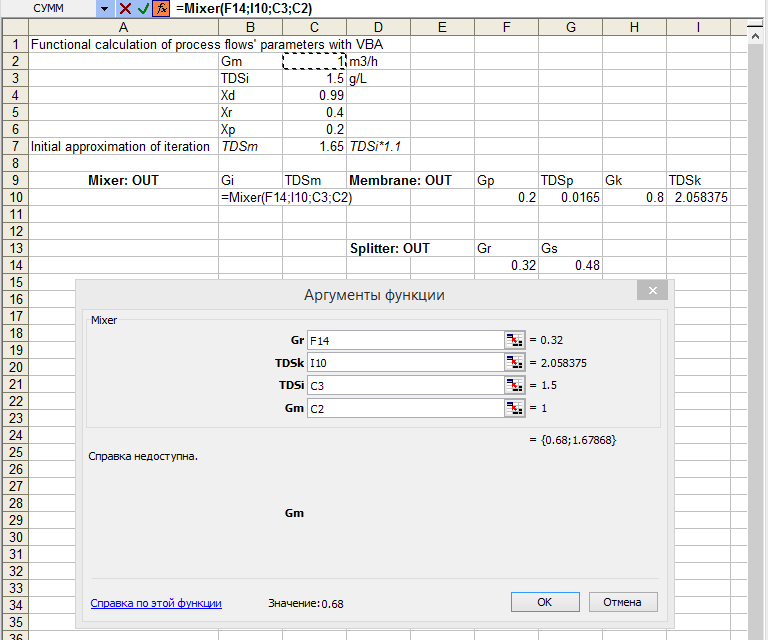Figure 3. Embedding a custom function in Excel

To start the calculation of the membrane, an initial approximation is necessary for the value of the water inlet TDS. At the end of the assembly, this value will be replaced by the calculated value from the function of the mixer. To use the iterative capabilities in Excel, one must enable the appropriate setting in the calculation parameters.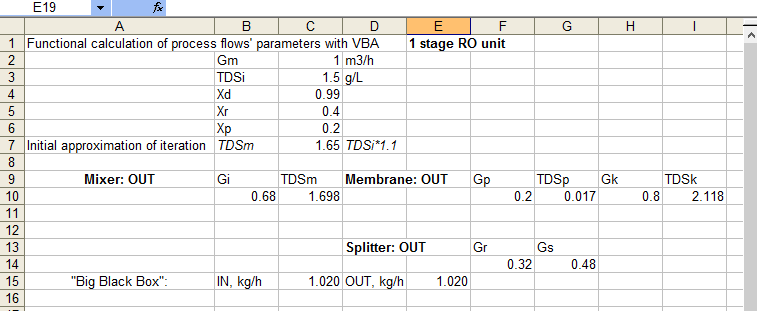Figure 4.  Results of calculation of single-stage installation

An interesting feature of such calculations in Excel is the loss of calculated values after opening a saved file (fig. 5).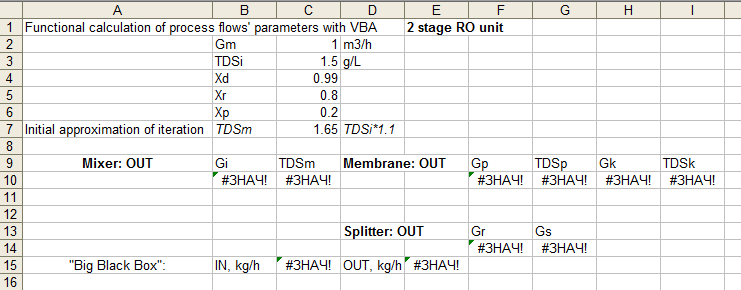Figure 5. The loss of calculated values after opening a saved file

To fix this error, one must delete one of the functions (not always obvious which one), and undo this action (fig.6 and fig.7).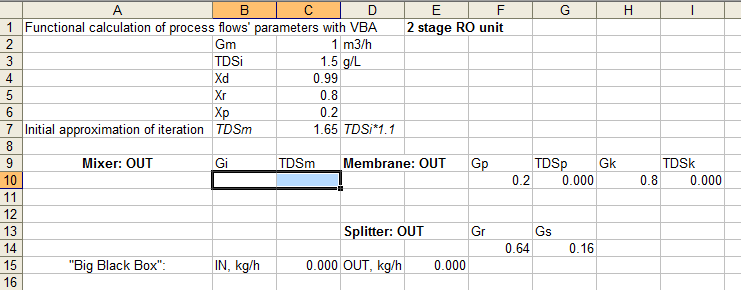Figure 6.  Deleting one of the functions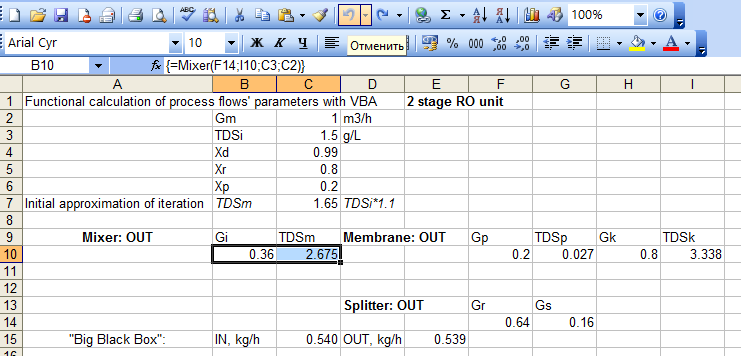Figure 7. Undoing delete action

To check the performed calculations, it is necessary to use the “big black box” principle, which allows estimating the flow rate at the input and output from the installation without taking into account the processes occurring inside the scheme. If these values do not match, then when making calculations, errors are made. The discrepancy between the input and output of the unit is less than 0.1% (1 g/hour from 540 g/hour), which indicates the correctness of the calculations performed.

Extending the calculation to a 2-stage RO scheme with recycling

Extending the calculation to a 2-stage scheme involves developing the function of the 2nd mixer (fig. 2) and using the same splitter and membrane functions, but with different input parameters (fig. 8). Obviously, for the second membrane, the input parameters will be the output parameters of the first one. The second mixer is “normal” in the sense that the parameters of its inputs (recycles after the 1st and 2nd splitter) are known, and the output parameters are calculated .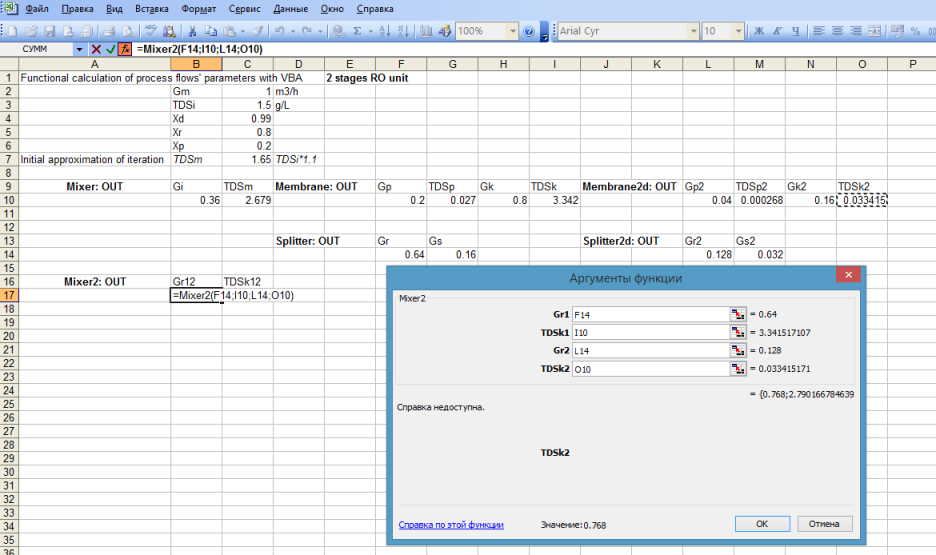Figure 8. Embedding additional custom functions

At the end of the calculation’s assembly, it is necessary to change the input parameters of the first mixer, the second input of which takes a mixture of two recycles instead of one (fig. 9). It should be noted that in practice the second recycle is usually not used in this way.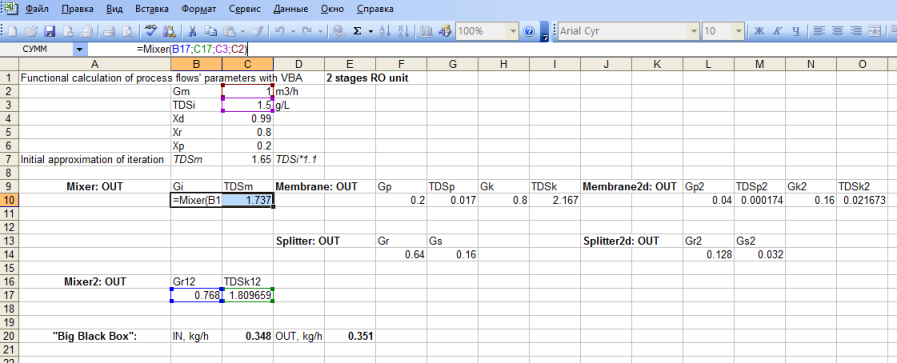Figure 9.  Changing the input parameters of the first mixer

The discrepancy between the input and output of the unit is less than 1% (3 g/hour from 350 g/hour), which indicates the correctness of the calculations performed.

It seems natural that by expanded the calculation scheme once it is possible to do this as many times as necessary with the same functions or with others.

Conclusions

A simple technique for calculating the parameters of technological processes of any complexity level is presented. For its implementation, the elementary knowledge of VBA language is required at the level of the organization of calculations of three types of apparatus and their combinations in user functions. The calculation of schemes with recycling flows requires the iteration possibility of Excel.
Such calculations lead to some easily solved problems associated with loss of calculation values after opening a saved file. At the same time, visualization of connections between functions on the Excel sheet, simplifies the organization of calculations, especially for beginners.
An important feature of the proposed approach is the possibility of independent creation of functions library by different developers. This is due to the functional approach applied with VBA in Excel environment, which ensures the reactivity of the calculations.

References
1. Functional programming. URL: https://en.wikipedia.org/wiki/Functional_programming.
2. Reactive programming. URL: https://en.wikipedia.org/wiki/Reactive_programming.
3. Бесков В. С. (сост.). Материальный баланс химико-технологической системы. Методическое пособие. – М.: РХТУ, 2003. – 64 с.